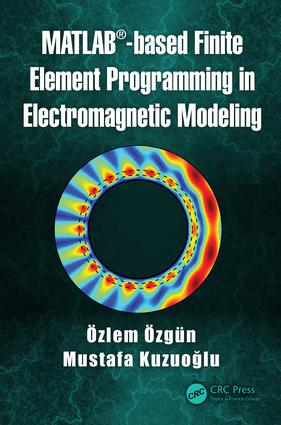MATLAB-based Finite Element Programming in Electromagnetic Modeling

1st Edition

CRC Press

428 pages | 21 Color Illus. | 157 B/W Illus.

Hardback: 9781498784078
pub: 2018-09-27
\$139.95
x
eBook (VitalSource) : 9780429457395
pub: 2018-10-10
from \$27.48

FREE Standard Shipping!

Description

This book is a self-contained, programming-oriented and learner-centered book on finite element method (FEM), with special emphasis given to developing MATLAB® programs for numerical modeling of electromagnetic boundary value problems. It provides a deep understanding and intuition of FEM programming by means of step-by-step MATLAB® programs with detailed descriptions, and eventually enabling the readers to modify, adapt and apply the provided programs and formulations to develop FEM codes for similar problems through various exercises. It starts with simple one-dimensional static and time-harmonic problems and extends the developed theory to more complex two- or three-dimensional problems. It supplies sufficient theoretical background on the topic, and it thoroughly covers all phases (pre-processing, main body and post-processing) in FEM. FEM formulations are obtained for boundary value problems governed by a partial differential equation that is expressed in terms of a generic unknown function, and then, these formulations are specialized to various electromagnetic applications together with a post-processing phase. Since the method is mostly described in a general context, readers from other disciplines can also use this book and easily adapt the provided codes to their engineering problems. After forming a solid background on the fundamentals of FEM by means of canonical problems, readers are guided to more advanced applications of FEM in electromagnetics through a survey chapter at the end of the book.

• Offers a self-contained and easy-to-understand introduction to the theory and programming of finite element method.
• Covers various applications in the field of static and time-harmonic electromagnetics.
• Includes one-, two- and three-dimensional finite element codes in MATLAB®.
• Enables readers to develop finite element programming skills through various MATLAB® codes and exercises.
• Promotes self-directed learning skills and provides an effective instruction tool.

Chapter 1 Introduction Chapter 2 MATLAB Primer Chapter 3 Mathematical Fundamentals of FEM Chapter 4 One-Dimensional Finite Element Analysis Chapter 5 Two-Dimensional Finite Element Analysis Chapter 6 Three-Dimensional Finite Element Analysis Chapter 7 Past and Present: A Selected Bibliography